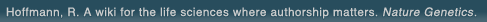# The world's first wiki where authorship really matters (Nature Genetics, 2008). Due credit and reputation for authors. Imagine a global collaborative knowledge base for original thoughts. Search thousands of articles and collaborate with scientists around the globe.

wikigene or wiki gene protein drug chemical gene disease author authorship tracking collaborative publishing evolutionary knowledge reputation system wiki2.0 global collaboration genes proteins drugs chemicals diseases compound
Hoffmann, R. A wiki for the life sciences where authorship matters. Nature Genetics (2008)# Carboplatin dosing: gender bias and inaccurate estimates of glomerular filtration rate.

The aim was to compare doses of carboplatin calculated using the Calvert formula and Chatelut formula and also to compare doses calculated using Calvert formula, modified with non-isotopic estimation of GFR, using the Cockcroft and Gault formula or the Jelliffe formula. For formulae comparison, doses were calculated to target an AUC of 7 mg/ml x min. When compared with the dose derived from the Calvert formula, the doses calculated in 122 adult cancer patients using the Chatelut formula were significantly higher for males and significantly lower for females. There was a statistically significant difference between the dose per kg calculated for males and females (P<0.0001). The mean percentage difference in dose calculated with substituted measures of renal function with the Cockcroft and Gault formula and Jelliffe formula was -8% (standard deviation (S.D.) 17%) and -14% (S.D. 16%), respectively. Further prospective evaluation of the Chatelut formula is required before it can be recommended for routine clinical application.

## References

1. Carboplatin dosing: gender bias and inaccurate estimates of glomerular filtration rate. Dooley, M.J., Poole, S.G., Rischin, D., Webster, L.K. Eur. J. Cancer (2002) [Pubmed]